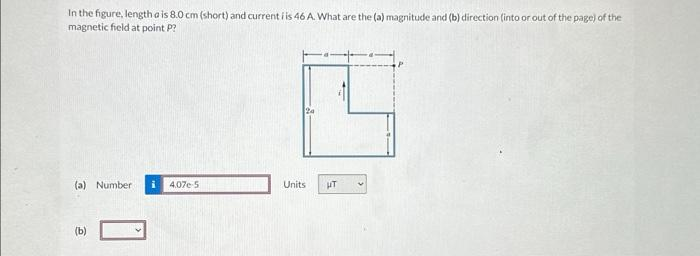# (Solved): In the figure, length a is 8.0cm (short) and current i is 46A. What are the (a) magnitude and ( ...In the figure, length is (short) and current i is . What are the (a) magnitude and (b) direction (into or out of the page) of the magnetic field at point P?

We have an Answer from Expert